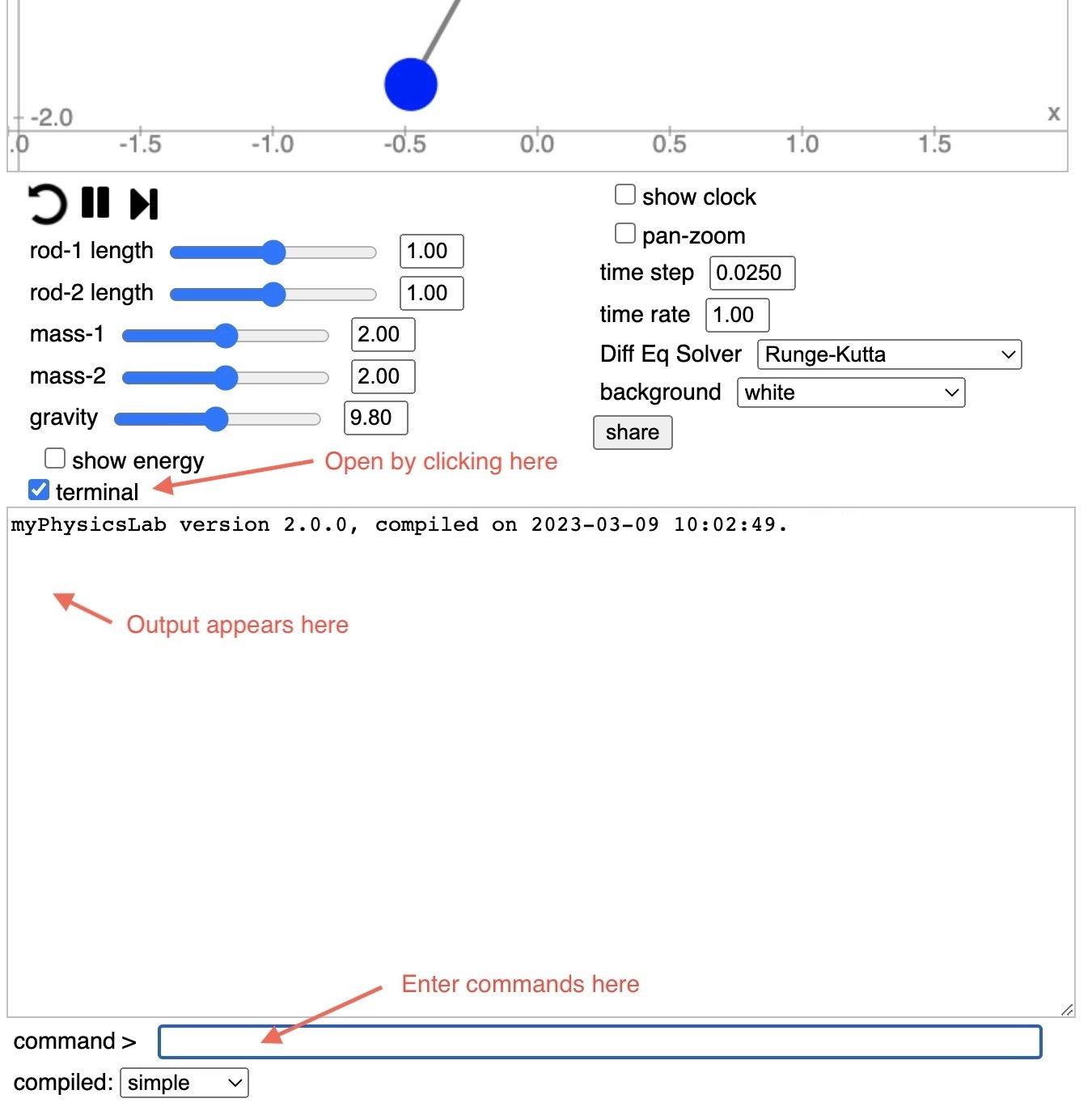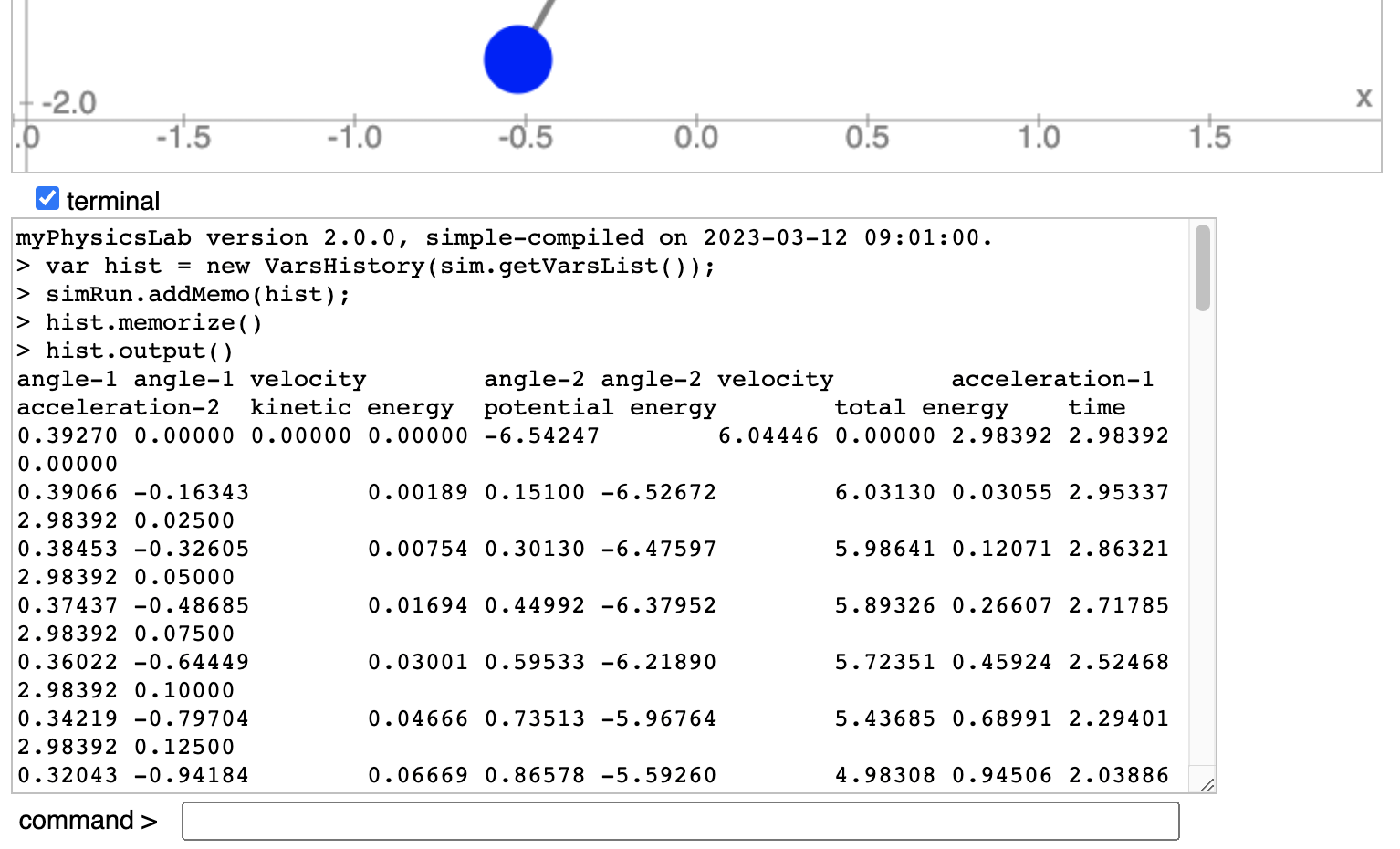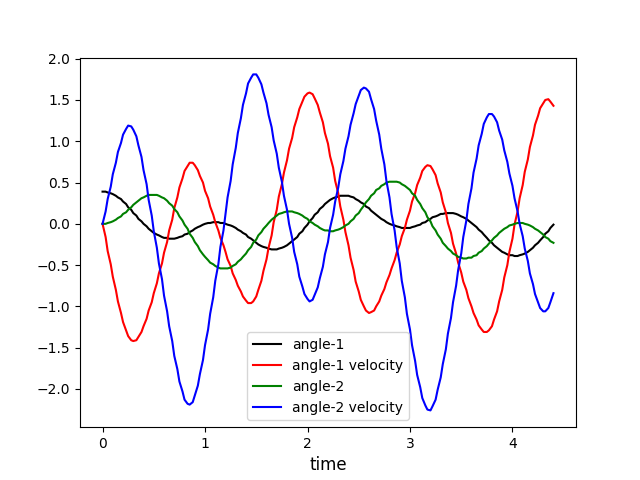myPhysicsLab Documentation

# Getting Numbers with VarsHistory

To get numeric data from a myPhysicsLab simulation using the VarsHistory class, follow the steps below.

Open the terminal window by clicking the “terminal” checkbox.Make sure you are using the simple-compiled version of the simulation. There should be a link to the simple-compiled version on the simulation’s web page, or find it in the set of simple-compiled applications.

Type `help` in the command box (and hit return of course) to see available Terminal commands.

Set your desired initial conditions on the simulation. Type or paste into the Terminal command box:

``````var hist = new VarsHistory(sim.getVarsList());
hist.memorize();
``````

To memorize the starting initial conditions, we call the `memorize()` function once before starting the simulation.

Run the simulation as long as you want. Click the “stop” button (or use a script to automatically stop at a certain time). In the Terminal command box type:

``````hist.output()
``````

This will print the data in Terminal output box. (Note: Don’t type a semi-colon “;” at the end of that line, the semi-colon suppresses the output in Terminal).

Here is how this would look in the Double Pendulum simulation.The first line contains the names of the variables. You can then select the text, copy and paste to a spreadsheet or text file. For example I was able to generate this graph from the Double Pendulum with a simple Python program.## Options Available for VarsHistory

The default separator between numbers is the tab character. To instead use comma separated values:

``````hist.setSeparator(', ');
``````

Use `setNumberFormat` to change the number formatting function. For example to show only two decimal places

``````hist.setNumberFormat((n) => n.toFixed(2));
``````

You can use Javascript’s Number.toExponential or one of the `myphysicslab.lab.Util` number format functions like Util.NF5E. The above example uses an arrow function but you can provide any function that takes one numeric argument and returns a string. Here is an equivalent non-arrow function:

``````hist.setNumberFormat(function(n) { return n.toFixed(2)});
``````

To change which variables are sampled or their order use `setVariables`. For example:

``````hist.setVariables([9,0,1,2,3])
``````

Find the index numbers of the variables by typing the command `names` into the Terminal command box. In the Double Pendulum simulation you would see this

``````SIM_VARS.ANGLE_1;
SIM_VARS.ANGLE_1_VELOCITY;
SIM_VARS.ANGLE_2;
SIM_VARS.ANGLE_2_VELOCITY;
SIM_VARS.ACCELERATION_1;
SIM_VARS.ACCELERATION_2;
SIM_VARS.KINETIC_ENERGY;
SIM_VARS.POTENTIAL_ENERGY;
SIM_VARS.TOTAL_ENERGY;
SIM_VARS.TIME
``````

So variable 0 is `ANGLE_1`, variable 1 is `ANGLE_1_VELOCITY`, etc. Another way to find index numbers of variables is with the command `prettyPrint(varsList)` which in the Double Pendulum produces

``````> prettyPrint(varsList)
VarsList{
name_: "SIM_VARS",
numVars: 10,
timeIdx_: 9,
history_: false,
(0) ANGLE_1: 0.39270,
(1) ANGLE_1_VELOCITY: 0.00000,
(2) ANGLE_2: 0.00000,
(3) ANGLE_2_VELOCITY: 0.00000,
(4) ACCELERATION_1: -6.54247,
(5) ACCELERATION_2: 6.04446,
(6) KINETIC_ENERGY: 0.00000,
(7) POTENTIAL_ENERGY: 2.98392,
(8) TOTAL_ENERGY: 2.98392,
(9) TIME: 0.00000,
``````

Note that calling `setVariables` erases all stored data from the VarsHistory. Therefore you should call `memorize` afterwards to store the initial conditions.

Here is a Terminal session that shows using several of these commands together in the Double Pendulum simulation.

``````> var hist = new VarsHistory(sim.getVarsList());
> hist.setNumberFormat((n) => n.toFixed(2));
> hist.setSeparator(',   ');
> hist.setVariables([9,0,1,2,3]);
> hist.memorize();
> hist.output()
time,   angle-1,   angle-1 velocity,   angle-2,   angle-2 velocity
0.00,   0.39,   0.00,   0.00,   0.00
0.03,   0.39,   -0.16,   0.00,   0.15
0.05,   0.38,   -0.33,   0.01,   0.30
0.08,   0.37,   -0.49,   0.02,   0.45
0.10,   0.36,   -0.64,   0.03,   0.60
0.13,   0.34,   -0.80,   0.05,   0.74
0.15,   0.32,   -0.94,   0.07,   0.87
0.18,   0.30,   -1.08,   0.09,   0.98
0.20,   0.27,   -1.19,   0.12,   1.08
0.23,   0.24,   -1.29,   0.14,   1.15
0.25,   0.20,   -1.36,   0.17,   1.19
``````